# Creating a Simple Formula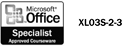A formula calculates values to return a result. On an Excel worksheet, you can create a formula using values (such as 147 or \$10.00), arithmetic operators (shown in the table), and cell references. An Excel formula always begins with the equal sign (=). The equal sign, when entered, automatically formats the cell as a formula entry. By default, only formula results are displayed in a cell , but you can change the view of the worksheet to display formulas instead of results.

#### Enter a Formula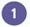Click the cell where you want to enter a formula.Type = (an equal sign). If you do not begin a formula with an equal sign, Excel will display, not calculate, the information you type.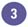Enter the first argument . An argument can be a number or a cell reference.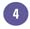Enter an arithmetic operator.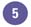Enter the next argument.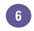Repeat steps 4 and 5 as needed to complete the formula.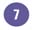Click the Enter button on the formula bar, or press Enter.

Notice that the result of the formula appears in the cell (if you select the cell, the formula itself appears on the formula bar).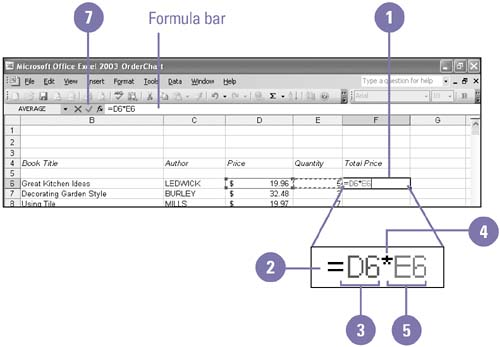Understanding Order of Precedence

Formulas containing more than one operator follow the order of precedence: exponentiation, multiplication and division, and then addition and subtraction. So, in the formula 5 + 2 * 3, Excel performs multiplication first and addition next for a result of 11. Excel calculates operations within parentheses first. The result of the formula (5 + 2) * 3 is 21.

## Did You Know?

The best way to start a formula is to have an argument . An argument is the cell references or values in a formula that contribute to the result. Each function uses function-specific arguments, which may include numeric values, text values, cell references, ranges of cells , and so on.

#### Display Formulas in CellsClick the Tools menu, and then click Options.Click the View tab.Click to select the Formulas check box.Click OK.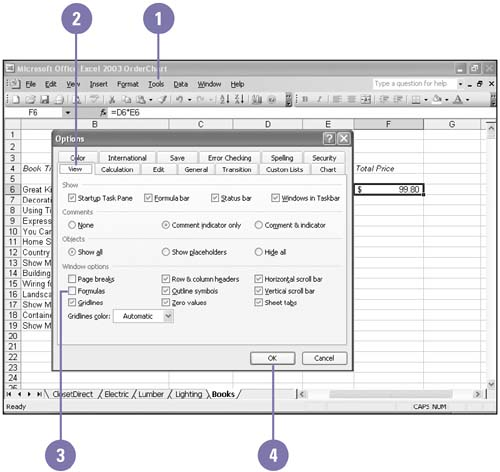## Did You Know?

Pointing to cells reduces errors . When building formulas, pointing to a cell rather than typing its address ensures that the correct cell is referenced.

You can select a cell to enter its address . To avoid careless typing mistakes, click a cell to insert its cell reference in a formula rather than typing its address.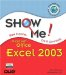Show Me Microsoft Office Excel 2003
ISBN: 0789730057
EAN: 2147483647
Year: 2002
Pages: 291